### TW University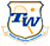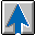Impact of a Tennis Ball with a Single Tennis String

Rod Cross, University of Sydney, Sydney, Australia
February 8, 2016

#### ABSTRACT

An experiment is described where a tennis ball was dropped vertically onto a single, horizontal tennis string. The objective was to determine the nature of the impact force between the ball and the string under conditions where the string could move freely, without being constrained by adjacent strings. The main questions of interest were whether the ball would grip or slide on the string and whether additional spin is generated when the string snaps back to its original position near the end of the impact. Results are presented on the measured rebound speed, angle and spin for cases where the ball was incident with or without spin. Video analysis of the string motion was used to help interpret the results, and a simplified theoretical bounce model is presented that is consistent with the experimental results.

Choose Movie:

Movie Screen 1 — The experiment in action. Three completely different bounces possible off a single string.
(Note: To see stringbed interaction, movies are best viewed frame by frame using keyboard arrow keys or movie controls.)

#### 1. INTRODUCTION

The modern game of tennis differs from that played in the wood racquet era, primarily because the ball is struck with considerably more spin. Part of the reason is that racquet heads are now larger, allowing players to swing up at the ball at a steeper angle and at higher speed without the ball striking the frame. A second reason is that most players now use low friction polyester strings rather than rough textured strings. The effect of low friction strings on ball spin is related to tangential motion of the strings within the string plane. Low friction strings move more freely across each other and can snap back to their original position while the ball is still on the strings, generating additional spin [1, 2]. An opposing effect is that the ball pushes the strings forward within the string plane so the normal reaction force shifts toward the front of the ball, thereby generating a counter torque that reduces the spin . The interaction between a tennis ball and the strings of a tennis racquet is a relatively complicated process since adjacent strings tend to act independently. All of the strings tend to move in unison in a direction perpendicular to the string plane, but motion in a direction parallel to the string plane can vary from one string to the next. Some strings move tangentially more than others, or in opposite directions, depending on whether the ball lands directly on one or more of the strings or whether it lands between two strings. In order to simplify the physics of the problem, a study was made of the interaction between a tennis ball and a single string. The resulting bounce is not representative of that between a ball and all the strings of a racquet but it does indicate how the ball can interact with any given string. The displacement of the string depends not only on the displacement of the ball but also on rotation of the ball during the impact. The latter effect is due to the fact that the the ball can grip the string, with the result that the string rotates in the same direction as the surface of the ball even if the centre of mass of the ball is moving in another direction. If a tennis ball collides head-on with a single tennis string, and if the ball is incident without spin, then the ball will rebound without spin back along the incident path. If the collision is not head-on then the rebound speed, spin and angle depend on the impact parameter, defined as the perpendicular distance between the string and the incident path of the ball centre of mass. The rebound parameters also depend on the spin of the incident ball. The results are not easy to predict and were therefore measured, for two different values of the incident spin. Measurements of string motion were also made to help interpret the results. String motion was easily observed and measured on video film since the displacement of the string during each impact was comparable to the radius of the ball. In most other collision experiments involving the impact of an object with a flexible surface, the behaviour of the surface itself is either very difficult to measure or interpret or can be inferred only indirectly from the behaviour of the impacting object.

#### 2. EXPERIMENTAL ARRANGEMENT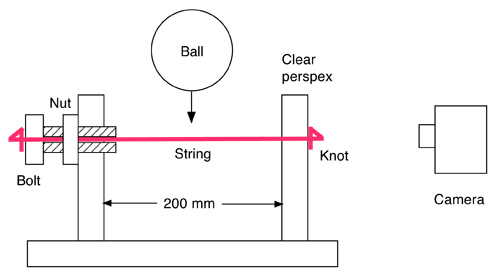Figure 1 — Experimental arrangement used to measure the impact of a tennis ball with a tennis string.

The arrangement used to measure low speed impacts of a tennis ball with a 1.25 mm diameter, polyester tennis string is shown in Fig. 1. The string was stretched between two vertical brackets located 200 mm apart, and tensioned with a nut and bolt located in the rear bracket. The tension was adjusted to a relatively low value, about 25 N, in order to increase the impact duration and to observe significant displacement of the string even for a low speed impact. During the impact itself, the string stretched by about 20 mm in the transverse direction, and the tension increased to a maximum estimated value of about 100 N. By comparison, the strings in a tennis racquet are normally woven, are tensioned to about 300 N, the tension rises to about 500 N during a firm impact with the ball, and the strings stretch by about 20 mm in a direction perpendicular to the string plane. The front bracket was made from clear perspex so that motion of both the ball and the string could be observed with a video camera viewing along the string. The ball was released from a fixed height to fall vertically through a distance of 86 cm onto the string, and to impact the string either "head-on" or towards one edge of the ball, as measured by the impact parameter, b, defined in Fig. 2.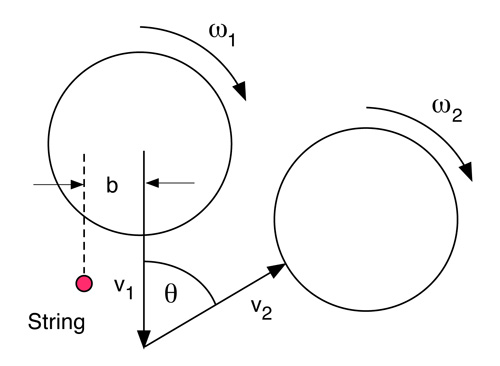Figure 2 — Camera view of an impact in Fig. 1, defining the main bounce parameters.

One set of measurements was taken without incident spin (ω1 = 0), and a second set of measurements was taken with ω1 = 70 +/- 2 rad/s, using a ribbon wrapped several times around the ball to impart spin to the ball as it fell. The impact was filmed either at 300 frames/s or 600 frames/s to capture motion of both the ball and the string during the impact and to measure the rebound angle, θ, the rebound speed, v2, and the rebound spin, ω2. Since the impact duration was typically about 17 ms, up to ten separate images of the ball and string were captured during each impact.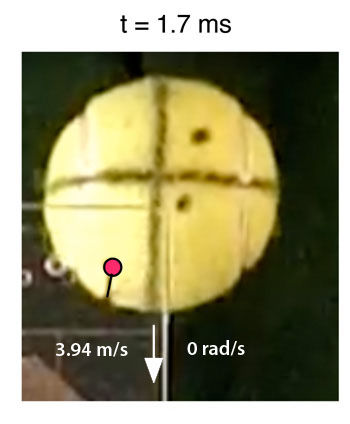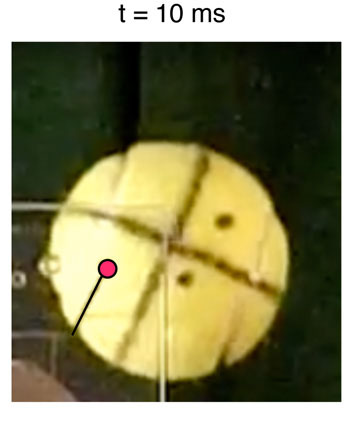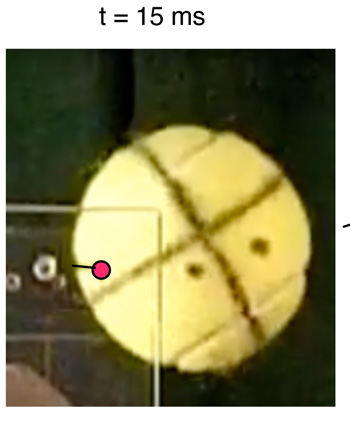Figure 3 — Motion of the ball and string when v1 = 3.94 m/s, b = 14 mm and ω1 = 0 rad/s. Both ends of the string remain fixed in time. The end facing the camera is shown as a large red dot. Initial impact occurred at t = 0. Equatorial lines drawn on the ball were used to measure ball rotation and to identify relative motion between the ball and the string.

A specific example is shown in Fig. 3, for a case where the ball was incident vertically without spin and bounced to the right spinning in a clockwise sense with ω2 = 119 rad/s. During the first 10 ms of the impact, the ball and the string both moved downwards by about 20 mm, by which time the ball was spinning rapidly due to the clockwise torque on the ball. The string rotated to the left since the ball gripped the string. Sliding and grip phases were easily identified by the presence or absence of relative motion of the string on the surface of the ball. In Fig. 3, the ball slipped slightly just before the end of the impact.

#### 3. BOUNCE RESULTS WITH ω1 = 0

Experimental results obtained with ω1 = 0 and v1 = 4.0 +/- 0.1 m/s are shown in Fig. 4 for impact parameters between -15 mm and +22 mm. Impacts with b < -15 mm were not examined since collisions were observed to be symmetrical about b = 0, as expected, when the ball is incident without spin. For impact parameters in the range -15 < b < +18 mm, the ball gripped the string throughout most of the impact, but when b > 20 mm, the ball slid on the string during the whole impact. For impacts with 18 < b < 20 mm, the ball gripped the string at the start of the impact and slid off the string towards the end of the impact. The sliding phase is analogous to a glancing angle collision of a ball on a flat, rigid surface where the resulting normal and friction forces are too small to reduce the tangential speed of the ball to zero at the contact surface.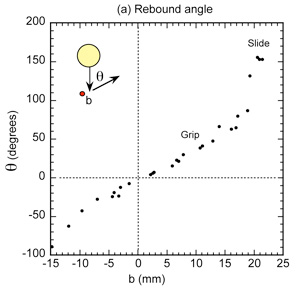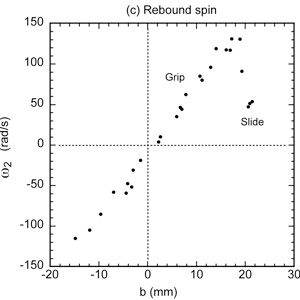Figure 4 — Results obtained when the ball was incident without spin at v1 = 4.0 +/- 0.1 m/s, showing (a) the rebound angle, θ, (b) the ratio of rebound speed to incident speed and (b) the rebound spin, ω2, all as a function of the impact parameter, b. Each data point corresponds to an individual bounce.

The grip phase results are also closely analogous to the bounce behaviour of a ball on a flat surface. For example, an impact with b = 0, corresponding to a head-on collision, results in a rebound with θ = 0, ω2 = 0 and where the rebound speed is a maximum. In the present case, v2/v1 had a maximum value of 0.81 +/- 0.01, representing the coefficient of restitution for a head-on impact. At larger values of b, the ball bounced away from the normal with increasing spin and decreasing speed as b increased, up to the point at which the ball began to slide on the string. The simultaneous increase in spin and decrease in rebound speed represents a partial conversion of translational energy into rotational energy. Analogous effects are observed when a ball bounces obliquely on a flat, rigid surface.

#### 4. BOUNCE RESULTS WHEN ω1 > 0

Significant differences are introduced when the incident ball is spinning, as shown in Fig. 5. Unlike the results for a ball incident without spin, the symmetry about b = 0 is broken. The ball bounces to the right when b = 0, with θ > 0, and it bounces without a significant change in spin. The video film provided a simple explanation for the behaviour when b = 0. The ball gripped the string on impact and deflected the string down and to the left since the bottom of the ball was moving down and rotating to the left. Consequently, the string exerted a force on the ball upwards and to the right. The force on the ball was directed towards the centre of the ball, so the torque about the centre of mass remained small and the change in ball spin was minimal.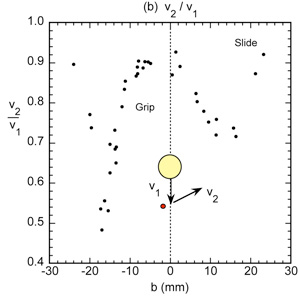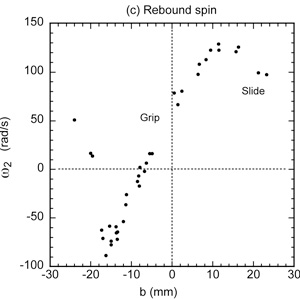Figure 5 —Results obtained with v1 = 3.7 +/- 0.15 m/s and ω1 = +70 +/- 4 rad/s.

For impacts near b = -12 mm, the ball bounced with θ close to zero and with a reversal in the spin direction. In that case, the string was deflected downwards during the first half of the impact, without a significant deflection to the left, since the large negative torque on the ball reduced the ball spin rapidly to zero. In addition, the ball slid on the string for the first few ms and did not move to the left even though the bottom of the ball was rotating to the left. Subsequently, the ball gripped the string and the string then moved along a nearly vertical path back to its equilibrium position. As a result, the ball bounced almost vertically and with a strong reversal in spin.

For other values of b, it was found that:

(a) when b < -18 mm, the ball slides on the string throughout the bounce,

(b) when -18 < b < -12 mm, the ball slides at the start of the bounce then grips the string, and may slide again at the end of the bounce.

(c) when b > -12 mm, the ball grips the string during the whole impact, even when incident with b > 15 mm where the ball is incident at a glancing angle. The latter effect was surprising but can be explained qualitatively in terms of acceleration of the ball. For example, if a mass is at rest on a stationary surface, and an external force is applied to the mass in a direction parallel to the surface, then static friction can allow the mass to remain at rest, provided that the external force is not too large. If the surface itself is accelerating in the direction of the external force, then the static friction required to prevent sliding is reduced. The static friction force could even be zero if the acceleration of the mass due to the external force is equal to the acceleration of the surface. Consequently, the ball can grip the string if the ball accelerates at about the same rate and in the same direction as the string would otherwise slide on a stationary ball.

#### 5. STRING MOTION

The behaviour of the ball during any given impact can be understood in terms of motion of the string. An example is shown in Fig. 6 where the ball was incident vertically without spin and with b = 14 mm. The same impact was shown previously in Fig. 3. The impact duration was 17 ms. Initially, the ball and the string both moved vertically downwards. The ball came to a stop in the vertical direction after 10 ms and then bounced to the right due to the force exerted by the string on the left side of the ball. The positive torque exerted by the string caused the ball to rotate clockwise. Since the ball gripped the string for most of the impact period, the string rotated to the left before returning to its initial position at the end of the impact. The force on the ball in Fig. 6 and the torque about its centre of mass was calculated from the stretch of the string. The force on the ball is given by F = kps where kp is the perpendicular stiffness of the string, s = √ (x2s + y2s) is the transverse displacement of the middle of the string, and xs and ys are the coordinates of the middle of the string as plotted in Fig. 6. kp is given by [5, 6]

(1)      kp = 4/L0 (T0 + 2ks2/L0)

where L0 is the initial length of the string, T0 is the initial string tension and k is the longitudinal stiffness of the string. The force on the ball is therefore a nonlinear function of s. For the experiment described above, L = 0.2 m, T = 25 +/- 1 N and k = 25 +/-2 kN/m, giving a perpendicular stiffness kp = 1000 N/m when s = 10 mm, or kp = 2500 N/m when s = 20 mm. The initial string tension and the perpendicular and longitudinal stiffness of the string were measured by hanging various weights on the string and measuring the transverse displacement of the middle of the string.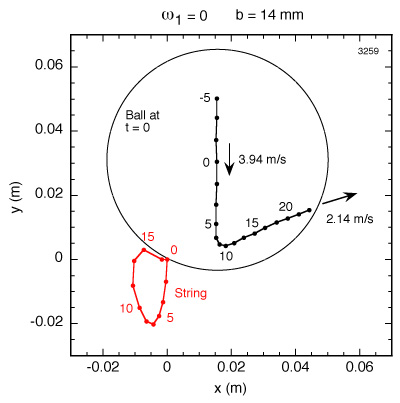Figure 6 —Result obtained when the ball was incident without spin at v1 = 3.94 m/s, with b = 14 mm, showing the positions of the centre of the ball and the middle of the string at intervals of 1/600 s (labelled at 5 ms intervals). The ends of the string remained fixed at x = 0, y = 0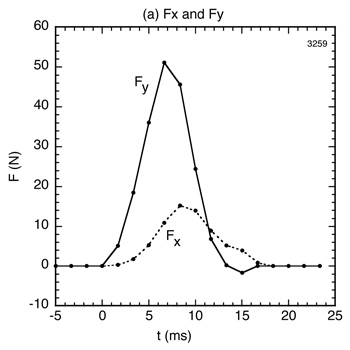Figure 7 —(a) The vertical (Fy) and horizontal (Fx) forces on the ball in Fig. 6, and (b) the torque about the centre of mass, calculated from the stretch of the string.

The x and y components of the string force were calculated from the relations Fx = kpxs and Fy = kpys, giving the results shown in Fig. 7(a). The torque, τ , about the centre of mass was calculated from the relation τ = Fy(xb – xs) – Fx(yb – ys), where xb, yb are the coordinates of the centre of mass, giving the result shown in Fig. 7(b).

The time integrals of F, Fy and τ were all consistent with the observed results for the ball where Int Fx dt = Mvx2 = 0.11 Ns, Int Fy dt = M(vy1 + vy2) = 0.27 Ns and Int τ dt = Icmω2 = 0.0041 Nms, where M is the mass of the ball, Icm is the moment of inertia about the centre of mass, and vx2 is the horizontal speed after the bounce. In theory, measurements of Fx, Fy and τ could also have been made by twice differentiating the linear and angular displacements of the ball as a function of time, but the digitized data were not sufficiently accurate to determine reliable values of the force components and the torque on the ball. The stretch of the string provided a direct and much more reliable estimate of those quantities.

#### 6. THEORETICAL MODEL OF THE BOUNCE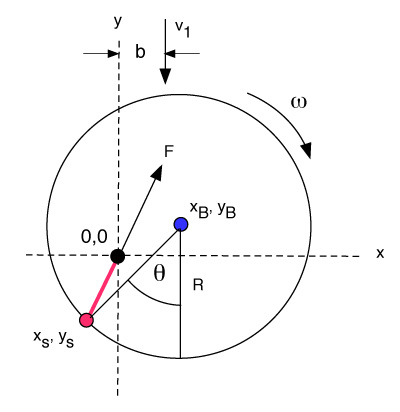Figure 8 —Geometry of vertical bounce of a ball on a horizontal string.

The bounce of the tennis ball can be modeled in terms of the geometry shown in Fig. 8. It is assumed that the ball remains in the x, y plane and the string is initially aligned along the z axis. The ball is incident vertically in the negative y direction at speed v1 with impact parameter b, so the centre of the ball is located at x = b prior to the collision and the string is located at x = 0, y = 0 prior to the collision. The coordinates of the ball centre of mass are denoted by xb, yb, both ends of the string remain fixed at x = 0, y = 0, and the middle of the string contacts the ball at coordinates xs, ys.

During the collision, the string stretches and exerts a force, F, on the ball in a direction from the contact point towards the (0, 0) origin, as indicated in Fig. 8. The torque acting on the ball causes the ball to rotate at angular velocity ω = dθ/dt , where θ is the angular displacement of the ball. In general, the string can slide on the ball or it can grip the ball, but we will assume that the string grips the ball at the start of the collision and continues to grip the ball until the end of the collision, or just prior to the end of the collision. The assumption of grip at the start is consistent with most of the observations and simplifies the analysis. The model is further simplified by ignoring energy loss in the ball.

The end of the collision is taken as the time at which the centre of the ball moves to a point located at distance R from the (0, 0) origin. Depending on the angular displacement of the ball, it is theoretically possible for ys to become positive near the end of the collision, but such a result is not consistent with experimental observations. Consequently, the string was allowed to slide on the ball near the end of the collision to ensure that ys remained zero rather than increasing to a positive value.

The equations of motion describing the ball are

(2)      Fx = kpxs = M (d2xb/dt2)

(3)      Fy = kpys = M d2yb/dt2)

(4)      τ = Fy(xb – xs) – Fx(yb – ys) = Icm (dω/dt)

where M is the mass of the ball, τ is the torque about the centre of mass and Icm = 0.55MR2 is the moment of inertia of the ball about its centre of mass. While the ball grips the string, the string coordinates are given by xs = xb – Rsinθ and ys = yb – Rcosθ.

Equations (1)-(4) were solved numerically to determine the motion of the ball and to determine the coordinates of the string at each time step. The results are shown in Fig. 9, assuming that v1 = 4 m/s, ω1 = 0 or 70 rad/s, the initial string tension T0 = 25 N and the 11 longitudinal string stiffness k = 25 kN/m. Since no energy loss mechanism was assumed, the peak v2/v1 ratio was 1.0 in Fig. 9(a). A slightly larger maximum v2/v1 ratio was calculated for the ball incident with spin, consistent with the experimental results shown in Figs. 4(b) and 5(b). That is, the maximum v2/v1 ratio was observed to increase when the ball was incident with spin.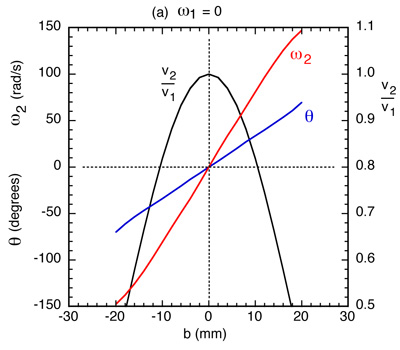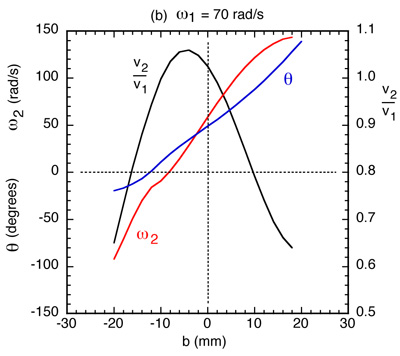Figure 9 —Model calculations for a ball incident vertically at 4.0 m/s on a horizontal tennis string with (a) ω1 = 0 and (b) ω1 = 70 rad/s.

At first sight, the result in Fig. 9(b) appears to indicate that the coefficient of restitution exceeded 1.0 for small negative values of b. However, the coefficient of restitution is conventionally defined in terms of the velocity components in a direction normal to the impact surface. In the present case, the impact surface rotates with the ball during the impact and is not well-defined. Instead, impacts with v2/v1 > 1 can be interpreted to mean that rotational energy was converted to translational energy during the impact since the ball bounced with ω2 < ω1.

The main features of the experimental results are reproduced by the theoretical model, and even the numerical results are broadly consistent with the results shown in Figs. 4 and 5. No attempt was made to reproduce the sliding phase results in Figs. 4 and 5 since glancing angle collisions with a tennis string are not commonly encountered in practice.

1. Nicolaides A, Elliott N, Kelley J, Pina o M, Allen T (2013) Effect of string bed pattern on ball spin generation from a tennis racket Sports Eng. 16:181-188
2. Haake SJ, Allen TB, Jones A, Spurr J, Goodwill S (2012) Effect of inter-string friction on tennis ball rebound. Proc Inst Mech Eng J J Eng Tribol 226:626-635
3. Kawazoe Y, Okimoto K (2008) Tennis top spin comparison between new, used and lubricated used strings by high speed video analysis with impact simulation. Theor Appl Mech Jpn 57:511-522
4. Lindsey C (2011) Spin and string pattern. The tennis warehouse. http://twu.tennis- warehouse.com/learning center/stringpattern.php. Accessed 1 Nov 2015
5. Cross R, Lindsey C (2013) Spin and string patterns old, new, and illegal. The tennis warehouse. http://twu.tennis-warehouse.com/ learning center/spinpatterns.php. Accessed 1 Nov 2015
6. R. Cross, Impact of a ball on a surface with tangential compliance, Am. J. Phys 78, 716-720 (2010).
7. R. Cross, Grip-slip behavior of a bouncing ball, Am. J. Phys. 70, 1093-1102 (2002).
8. R. Cross, C. Lindsey, D.Andruczyk, Laboratory testing of tennis strings, Sports Eng. 3, 219-230 (2000).
9. H. Brody, R. Cross, C. Lindsey, The Physics and Technology of Tennis, Racquet Tech Publishing, Solana Beach 2002 Chapter 13.